Archived | Using Keras and TensorFlow for anomaly detection

Archived content

Archive date: 2021-06-09

This content is no longer being updated or maintained. The content is provided “as is.” Given the rapid evolution of technology, some content, steps, or illustrations may have changed.

Note: IBM Data Science Experience (DSX) is now IBM Watson Studio. Although the name has changed and some images may show the previous name, the steps and processes in this tutorial will still work.

After introducing you to deep learning and long-short term memory (LSTM) networks, I showed you how to generate data for anomaly detection. Now, in this tutorial, I explain how to create a deep learning neural network for anomaly detection using Keras and TensorFlow.

As a reminder, our task is to detect anomalies in vibration (accelerometer) sensor data in a bearing as shown in Accelerometer sensor on a bearing records vibrations on each of the three geometrical axes x, y, and z.

Accelerometer sensor on a bearing records vibrations on each of the three geometrical axes x, y, and zWhen talking about deep learning a lot of people often talk about libraries such as TensorFlow and PyTorch. Those are great tools, but in my opinion provide relatively low-level support, meaning you have to think a lot about linear algebra and the shapes of matrices. Keras, on the other hand, is a high-level abstraction layer on top of popular deep learning frameworks such as TensorFlow and Microsoft Cognitive Toolkit—previously known as CNTK; Keras not only uses those frameworks as execution engines to do the math, but it is also can export the deep learning models so that other frameworks can pick them up. And because we’ve introduced Deeplearning4j and SystemML already in this series, I’m happy to tell you that both frameworks can read and execute on Keras models. Isn’t that great? You can do the fast prototyping in Keras and then scale out on Apache Spark using Deeplearning4j or SystemML as an execution framework for your Keras models. Finally, for completeness, there exists frameworks like TensorFrames and TensorSpark to directly bring TensorFlow to Apache Spark, but this is beyond this article.

What you’ll need to build your app

• An IBM Cloud account. (Sign up for an IBM Cloud account.)
• An IBM Watson Studio account. You can use the IBM ID you’ve created while registering for the IBM Cloud Account.

Before we talk about the deep learning use case, spend some time setting up your development environment. We use a Jupyter Notebook running inside Watson Studio.

We’ll start with a little example of two “pickled” data sets (the pickle interface is the serialization and deserialization framework in Python) – one containing healthy data and one containing broken data to develop the neural network.

Afterward we’ll turn this notebook into a real-time anomaly detector accessing data directly from an MQTT topic by using the IBM Watson IoT Platform.

2. Import a Jupyter notebook that contains all the necessary code into Watson Studio.

1. In the top right, click Create new > Notebook.

Note: If you have an existing account, from the upper left click IBM Watson Studio to open the dashboard, and then click Get started. From there you can click New notebook.

2. Click From URL, in the Name field add a name, and in the Notebook URL field, paste the following URL: https://raw.githubusercontent.com/romeokienzler/developerWorks/master/WatsonIoTPlatformKerasTFLSTM.ipynb
3. Click Create Notebook.

In theory, you could already start from here, but let me introduce Keras and TensorFlow a bit and then walk you through the code.

What is TensorFlow?

Google released TensorFlow under the Apache 2.0 open source license in 2015.

TensorFlow has two components: an engine executing linear algebra operations on a computation graphand some sort of interface to define and execute the graph. The engine in TensorFlow is written in C++, in contrast to SystemML where the engine is written in JVM languages. Although different language bindings for TensorFlow exist, the most prominent one is Python.

The engine of TensorFlow can run on CPUs, GPUs, TPUs, and for mobile and embedded devices there is TensorFlow Lite.There are three components of the engine:

• A client component that creates and takes your computational execution graph and submits it to the master component.
• A master component that parses the computational execution graph and distributes it to worker components.
• Worker components that execute parts of the computational execution graph.

Worker components can make use of specialized hardware like GPUs and TPUs. Distributed TensorFlow can run on multiple machines, but this is not covered in this article because we can use Deeplearning4j and Apache SystemML for distributed processing on Apache Spark without the need to install distributed TensorFlow.

In other words, TensorFlow is nothing more than a domain specific language (DSL) expressed in Python to define a computational execution graph for linear algebra operations and a corresponding (parallel) execution engine for running an optimized version of it.

What is Keras?

You might have noticed that TensorFlow can get to low-level linear algebra sometimes, and the code doesn’t even look nice from a mathematician’s perspective. Therefore, I suggest using Keras wherever possible. This blog post titled Keras as a simplified interface to TensorFlow: tutorial is a nice introduction to Keras. I will explain Keras based on this blog post during my walk-through of the code in this tutorial.

Create a Keras neural network for anomaly detection

We need to build something useful in Keras using TensorFlow on Watson Studio with a generated data set. (Remember, we used a Lorenz Attractor model to get simulated real-time vibration sensor data in a bearing. We need to get that data to the IBM Cloud platform. See the tutorial on how to generate data for anomaly detection.) When we set up our development environment we imported the WatsonIoTPlatformKerasTFLSTM Notebook and we’ll look at it now. Note: If you closed Watson Studio, open the WatsonIoTPlatformKerasTFLSTM notebook you previously imported.

Install and import the dependencies

Initially, we make sure that all dependencies are installed.

import pip

try:
__import__('keras')
except ImportError:
pip.main(['install', 'keras'])

try:
__import__('h5py')
except ImportError:
pip.main(['install', 'h5py'])

try:
__import__('ibmiotf')
except ImportError:
pip.main(['install', 'ibmiotf'])

Next, we review the notebook and the list of dependencies. (Note: Keras is already preinstalled in Watson Studio.)

import numpy as np
from numpy import concatenate
from matplotlib import pyplot
from pandas import DataFrame
from pandas import concat
import sklearn
from  sklearn.preprocessing import MinMaxScaler
from sklearn.preprocessing import MinMaxScaler
from sklearn.preprocessing import LabelEncoder
from sklearn.metrics import mean_squared_error
from keras.models import Sequential
from keras.layers import Dense
from keras.layers import LSTM
from keras.callbacks import Callback
from keras.models import Sequential
from keras.layers import LSTM, Dense, Activation
import pickle
import matplotlib.pyplot as plt
from mpl_toolkits.mplot3d import Axes3D
import ibmiotf.application
from Queue import Queue
%matplotlib inline

Next, we need to download two data files that contain some samples of broken and healthy data.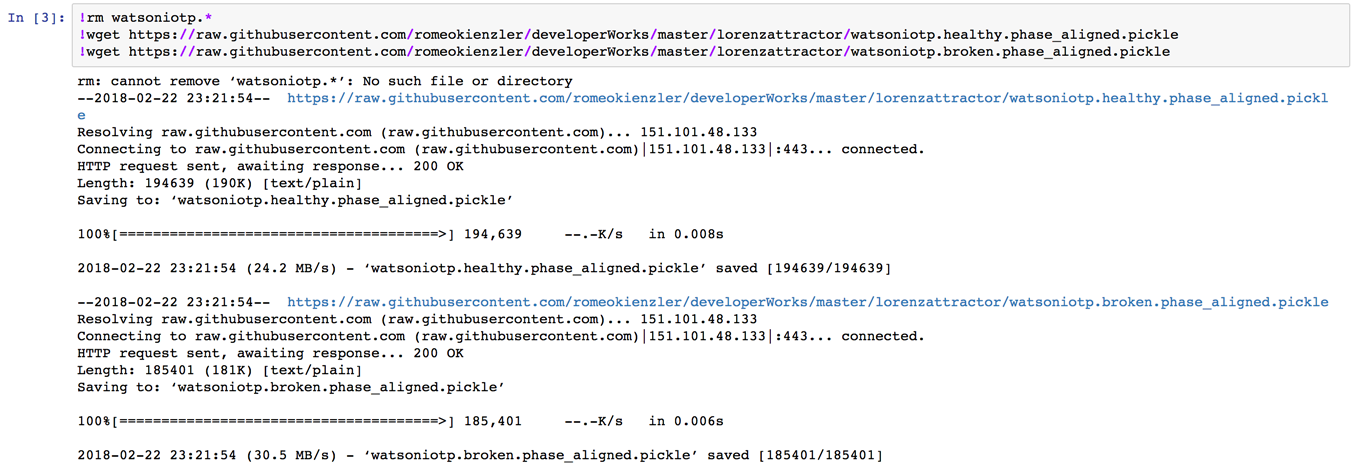!rm watsoniotp.*
!wget https://raw.githubusercontent.com/romeokienzler/developerWorks/master/lorenzattractor/watsoniotp.healthy.phase_aligned.pickle
!wget https://raw.githubusercontent.com/romeokienzler/developerWorks/master/lorenzattractor/watsoniotp.broken.phase_aligned.pickle

Deserialize the two numpy arrays

The two data files are serialized numpy arrays using the Python pickle library. We need to de-serialize (rematerialize) those two arrays from the files.

Set the correct shape for the data

To make sure the shapes are correct we set them both to have three columns and 3000 rows.

data_healthy = data_healthy.reshape(3000,3)
data_broken = data_broken.reshape(3000,3)

Visually inspect the data

First, we have a look at the healthy data. Notice that while this system oscillates between two semi-stable states, it is hard to identify any regular patterns.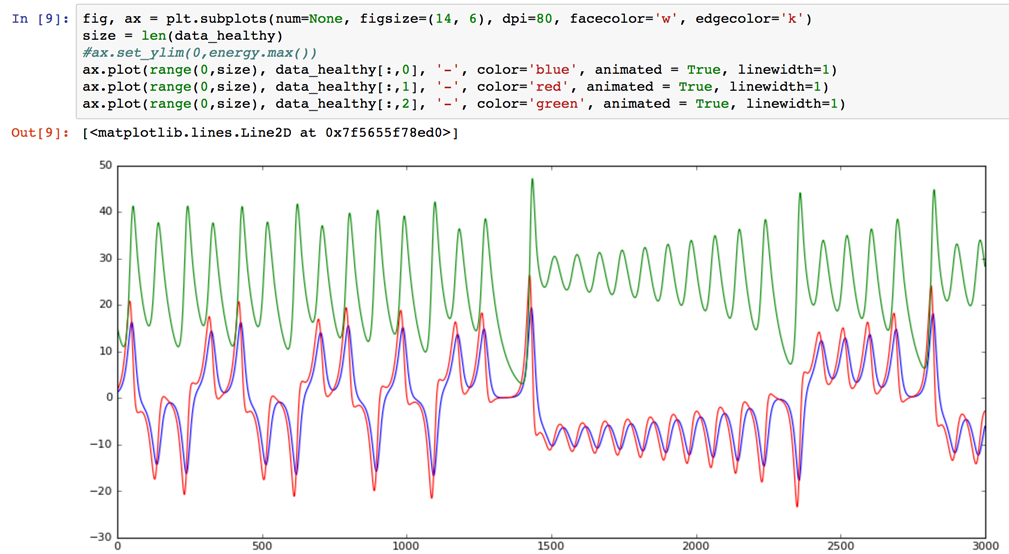fig, ax = plt.subplots(num=None, figsize=(14, 6), dpi=80, facecolor='w', edgecolor='k')
size = len(data_healthy)
#ax.set_ylim(0,energy.max())
ax.plot(range(0,size), data_healthy[:,0], '-', color='blue', animated = True, linewidth=1)
ax.plot(range(0,size), data_healthy[:,1], '-', color='red', animated = True, linewidth=1)
ax.plot(range(0,size), data_healthy[:,2], '-', color='green', animated = True, linewidth=1)

Next, run the cell look at the same chart after we’ve switched the test data generator to a broken state. The obvious result is that we see much more energy in the system. The peaks are exceeding 200 in contrast to the healthy state which never went over 50. Also, in my opinion, the frequency content of the second signal is higher.fig, ax = plt.subplots(num=None, figsize=(14, 6), dpi=80, facecolor='w', edgecolor='k')
size = len(data_healthy)
#ax.set_ylim(0,energy.max())
ax.plot(range(0,size), data_broken[:,0], '-', color='blue', animated = True, linewidth=1)
ax.plot(range(0,size), data_broken[:,1], '-', color='red', animated = True, linewidth=1)
ax.plot(range(0,size), data_broken[:,2], '-', color='green', animated = True, linewidth=1)

Let’s confirm the frequency of the second signal is higher by transforming the signal from the time to the frequency domain.

data_healthy_fft = np.fft.fft(data_healthy)
data_broken_fft = np.fft.fft(data_broken)

The chart now contains the frequencies of the healthy signal.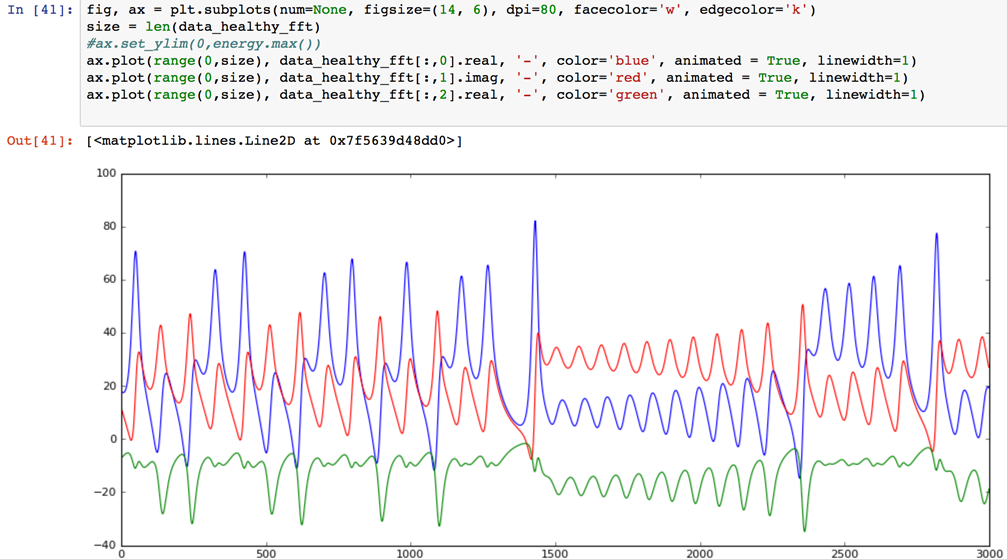fig, ax = plt.subplots(num=None, figsize=(14, 6), dpi=80, facecolor='w', edgecolor='k')
size = len(data_healthy_fft)
ax.plot(range(0,size), data_healthy_fft[:,0].real, '-', color='blue', animated = True, linewidth=1)
ax.plot(range(0,size), data_healthy_fft[:,1].imag, '-', color='red', animated = True, linewidth=1)
ax.plot(range(0,size), data_healthy_fft[:,2].real, '-', color='green', animated = True, linewidth=1)

Note: We are plotting the imaginary part of the red dimension to see three lines because two dimensions on this dataset are completely overlapping in frequency and the real part is zero. Remember, the way FFT (fast Fournier transform) works is retuning the sine components in the real domain and the cosine components in the imaginary domain. Just a hack mathematicians use to return a tuple of vectors.

Let’s contrast this healthy data with the broken signal. As expected, there are a lot more frequencies present in the broken signal.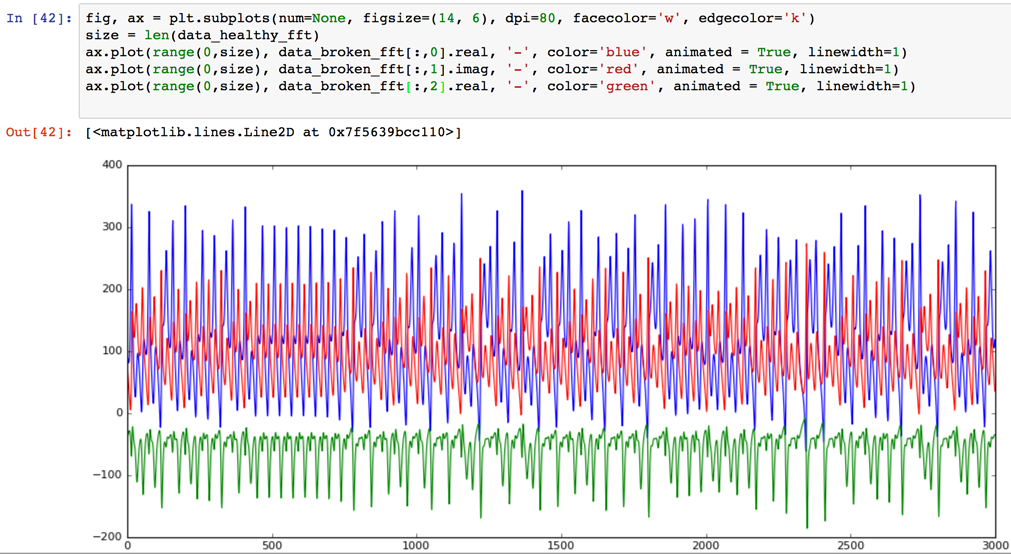fig, ax = plt.subplots(num=None, figsize=(14, 6), dpi=80, facecolor='w', edgecolor='k')
size = len(data_healthy_fft)
ax.plot(range(0,size), data_broken_fft[:,0].real, '-', color='blue', animated = True, linewidth=1)
ax.plot(range(0,size), data_broken_fft[:,1].imag, '-', color='red', animated = True, linewidth=1)
ax.plot(range(0,size), data_broken_fft[:,2].real, '-', color='green', animated = True, linewidth=1)

Create unsupervised machine learning

We now have enough evidence to construct an anomaly detector based on supervised machine learning (with a state-of-the-art model like a gradient boosted tree). But we want unsupervised machine learning because we have no idea which parts of the signal are normal and which are not.

A simple approach to unsupervised machine learning is to feed those 3,000 frequency bands into an ordinary feed-forward neural network. Remember, DFT (discrete Fourier transform) returns as many frequency bands as we have samples in the signal, and because we are sampling with 100 Hz for 30 seconds from the physical model this is also the number of frequency bands.

With this approach we have transformed our three-dimensional input data (the three accelerometer axes we are measuring) into a 9,000 dimensional data set (the 3,000 frequency bands per accelerometer axis). This is our new 9,000 dimensional input feature space. We can use the 9,000 dimensional input space to train a feed-forward neural network. Our hidden layer in the feed-forward neural network has only 100 neurons (instead of the 9,000 we have in the input and output layer). This is called a bottleneck and turns our neural network into an autoencoder.

We train the neural network by assigning the inputs on the input and output layers. The neural network will learn to reconstruct the input on the output. But the neural network has to learn the reconstruction going through the 100 neuron hidden-layer bottleneck. This way we prevent the neural network from learning about any noise or irrelevant data. We will skip this step here because the performance of such an anomaly detector is usually quite low. You can see an implementation of this in my last article on Apache SystemML where I’ve implemented a feed-forward neural network auto-encoder.

When working with neural networks it is always good to scale data to a range between zero and one. Because we are planning to turn this notebook into a real-time anomaly detector for IoT sensor date we are defining a function instead of transforming the data so that we can make use of the transformer at later stage.

def scaleData(data):
# normalize features
scaler = MinMaxScaler(feature_range=(0, 1))
return scaler.fit_transform(data)

We next scale our two sample data arrays.

data_healthy_scaled = scaleData(data_healthy)
data_broken_scaled = scaleData(data_broken)

Improve anomaly detection by adding LSTM layers

We can outperform state-of-the-art time series anomaly detection algorithms and feed-forward neural networks by using long-short term memory (LSTM) networks.

Based on recent research (the 2012 Stanford publication titled Deep Learning for Time Series Modeling by Enzo Busseti, Ian Osband, and Scott Wong), we will skip experimenting with deep feed-forward neural networks and directly jump to experimenting with a deep, recurrent neural network because it uses LSTM layers. Using LSTM layers is a way to introduce memory to neural networks that makes them ideal for analyzing time-series and sequence data.

To get started let’s reshape our data a bit because LSTMs want their input to contain windows of times.

timesteps = 10
dim = 3
samples = 3000
data_healthy_scaled_reshaped = data_healthy_scaled
#reshape to (300,10,3)
data_healthy_scaled_reshaped.shape = (samples/timesteps,timesteps,dim)

This way instead of 3000 samples per dimension (per vibration axis) we have 300 batches of length 10. What we want to do is given the last 10 time steps of the signal predict the future 10.

Let’s walk through that code a bit. The first thing we need to do in Keras is create a little callback function which informs us about the loss during training. The loss is basically a measure how well the neural network fits to the data. The lower the better (unless we are not overfitting).

losses = []

def handleLoss(loss):
global losses
losses+=[loss]
print loss

class LossHistory(Callback):
def on_train_begin(self, logs={}):
self.losses = []

def on_batch_end(self, batch, logs={}):
self.losses.append(logs.get('loss'))
handleLoss(logs.get('loss'))

It gets interesting because we are now defining the neural network topology.

# design network

model = Sequential()

def train(data):
data.shape = (300, 10, 3)
model.fit(data, data, epochs=50, batch_size=72, validation_data=(data, data), verbose=0, shuffle=False,callbacks=[LossHistory()])
data.shape = (3000, 3)

def score(data):
data.shape = (300, 10, 3)
yhat =  model.predict(data)
yhat.shape = (3000, 3)
return yhat

Let’s walk through this code. First, we create an instance of a Sequential model. This allows us to add layers to the model as we go.

model = Sequential()

Then, we add an LSTM layer as first layer with 50 internal neurons, input shape of 10 by three, and we want the layer to return a sequence of the predicted future time steps.

We do this eleven times, making it an eleven layers deep LSTM neural network. Why did I choose eleven? This is part of the black magic. The more layers you add the more accurate the predictions are (up to a certain point), but the more compute power is necessary. In most cases, tuning the neural network topology and (hyper)-parameters is considered “black magic” or “trial-and-error.”

To get back to normal, we finalize with a normal, fully connected feed-forward layer to bring down the dimensions to three again.

Finally, we compile the model with two parameters:

• loss=mae, which means that the training error during training and validation is measured using the “mean absolute error” measure.

For convenience we create two functions for training and scoring. (Note: We provide the same data as input and output, because we are creating an auto-encoder.)

model.fit(data, data, epochs=50, batch_size=72,...

Let’s do the test and train the neural network twenty times with healthy data and one time with broken data. Remember that your callback function handleLoss adds the trajectory of losses (time-series) to an array which we can plot. Therefore, we should see a spike whenever the healthy data pre-trained neural network sees broken data. Not because it knows what healthy or broken is, but because it tells us that it is unlikely that this time-series pattern the neural network currently sees has been seen before.

for i in range(20):

print "----------------"
train(data_healthy_scaled)
yhat_healthy = score(data_healthy_scaled)
yhat_broken = score(data_broken_scaled)
data_healthy_scaled.shape = (3000, 3)
data_broken_scaled.shape = (3000, 3)

print "----------------broken"
train(data_broken_scaled)
yhat_healthy = score(data_healthy_scaled)
yhat_broken = score(data_broken_scaled)
data_healthy_scaled.shape = (3000, 3)
data_broken_scaled.shape = (3000, 3)

After the training is finished we see this by the absence of the star symbol in the cell caption. It is replaced by a number indicating the execution is finished.

for i in range(20):

Let’s plot the losses to see if we can detect a spike on the abnormal (broken) data.fig, ax = plt.subplots(num=None, figsize=(14, 6), dpi=80, facecolor='w', edgecolor='k')
size = len(data_healthy_fft)
#ax.set_ylim(0,energy.max())
ax.plot(range(0,len(losses)), losses, '-', color='blue', animated = True, linewidth=1)

And here it is. On time step 5000 we can clearly see that something is going on. Note that the initial loss was higher, but this is because initially the weights of the neural network have been initialized randomly, therefore leading to bad predictions.

Analyze the data in real-time with the IBM Watson IoT Platform using MQTT

The last step is to hook this anomaly detector up to the IBM Watson IoT Platform using MQTT to analyze data in real-time. To hook-up our neural network to the platform is straightforward. The values you need are highlighted in IBM Cloud app credentials: org, apiKey, and apiToken. These credentials were generated when you created an IBM Cloud app using the Internet of Things Platform Starter.

Note: Refer to the Generating data for anomaly detection article for details on this process.

IBM Cloud app credentials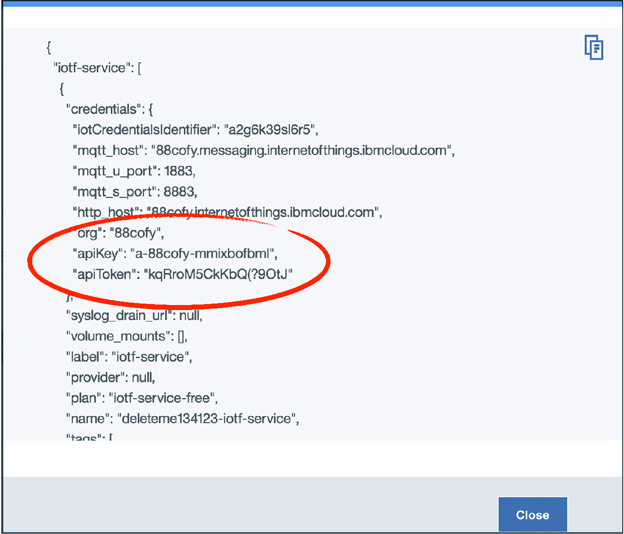Let’s connect to the MQTT message broker directly from the notebook.

options = {"org": "mh741h", "id": "anything", "auth-method": "apikey", "auth-key": "a-mh741h-hwv6qlpmsv", "auth-token": "zBcbK&rKTfbW*OfneW"}
client = ibmiotf.application.Client(options)
client.connect()

Perform streaming analysis by creating a count-based tumbling window

We need to create a window for our data (actually we create a Python queue).

q = Queue(7000)

We are working on count-based tumbling windows of size 3000. We can store more than two such windows in the queue until we prevent the Watson IoT Platform’s MQTT message broker to accept new messages. Nevertheless, we need to make sure we are running fast enough to cope with the data arrival rate, otherwise the system will eventually trash. We subscribe to data coming fromthe MQTT message broker and define a call-back function which puts the data into our queue.

def myEventCallback(event):
sample = event.data
point = [sample["x"], sample["y"],sample["z"]]
q.put(point)

client.deviceEventCallback = myEventCallback
client.subscribeToDeviceEvents("0.16.2", "lorenz", "osc")

Then we define a function which gets called whenever a count based tumbling window of 3000 samples is full.

def doNN(data):
data_scaled = scaleData(data)
train(data_scaled)
yhat = score(data_scaled)
data_scaled.shape = (3000, 3)

Note that we are scaling the data before we call the “train” function which trains our anomaly detector with data (and here we don’t know whether it is healthy or broken data).

We want to send the current loss during training (remember, we are always training) back to the platform so that we are creating a real-time anomaly score.

def handleLoss(loss):
myData={'loss' : str(loss)}
client.publishEvent("0.16.2", "lorenz", "status", "json", myData)

Here it became handy that we can override the handleLoss function to add that functionality.

Create a continuous application loop for training

Now we need to start an infinitely running event loop which does the following:

1. Loop infinitely

1. Loop over the queue if it is not empty

1. If there is data, put it to a temporary array
2. If the count-based sliding window is full then

1. Send the window to the neural network
2. Empty the array

import numpy as np
while True:
while not q.empty():
point = q.get()
try:
data
except NameError:
data = np.array(point)
else:
data = np.append(data,point)
if data.size>=9000:
data = np.reshape(data,(3000,3))
print data
doNN(data)
del data

Because the actual training loss is sent back to the IBM Watson IoT Platform in real-time we can create a little dashboard to visualize the vibration sensor data together with the anomaly score in real-time (creation of this dashboard is not part of this article).Conclusion

This completes our third deep-learning tutorial for IoT time-series data and concludes the series. We’ve learned how TensorFlow accelerates linear algebra operations by optimizing executions and how Keras provides an accessible framework on top of TensorFlow.

Finally, we’ve shown that even an LSTM network can outperform state-of-the-art anomaly detection algorithms on time-series sensor data – or any type of sequence data in general.

Acknowledgements

I’m deeply thankful to Michelle Corbin and Gina Caldanaro – two fantastic editors – for working with me on this series.

Legend